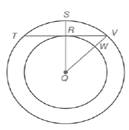Chapter 6.1, Problem 17E### Elementary Geometry for College St...

6th Edition
Daniel C. Alexander + 1 other
ISBN: 9781285195698

#### Solutions

Chapter
Section### Elementary Geometry for College St...

6th Edition
Daniel C. Alexander + 1 other
ISBN: 9781285195698
Textbook Problem
1 views

# Given: Concentric circles with center Q T V = 8  and  V W = 2 R Q ¯ ⊥ T V ¯Exercises 16, 17 Find: R Q (HINT: Let R Q = x . )

To determine

To calculate:

The value of RQ by using the provided figure.

Explanation

Given:

The concentric circles with centre Q, RQ¯TV¯, TV=8 and VW=2

Postulate used:

A radius that is perpendicular to a chord bisects the chord.

Concentric circle:

Concentric circle are coplanar circles that have a common centre.

Since, a radius is a line segment that joins the centre of the circle to a point on a circle.

Calculation:

Let, RQ=x

Since, RQ¯TV¯ then RT=TV where TV=8 and VW=2.

Now, RV=12TV then we obtain

### Still sussing out bartleby?

Check out a sample textbook solution.

See a sample solution

#### The Solution to Your Study Problems

Bartleby provides explanations to thousands of textbook problems written by our experts, many with advanced degrees!

Get Started

#### Evaluate limx01+tanx1+sinxx3.

Single Variable Calculus: Early Transcendentals

#### n=12(14)n converges to: a) 94 b) 2 c) 23 d) the series diverges

Study Guide for Stewart's Single Variable Calculus: Early Transcendentals, 8th

#### True or False:

Study Guide for Stewart's Multivariable Calculus, 8th